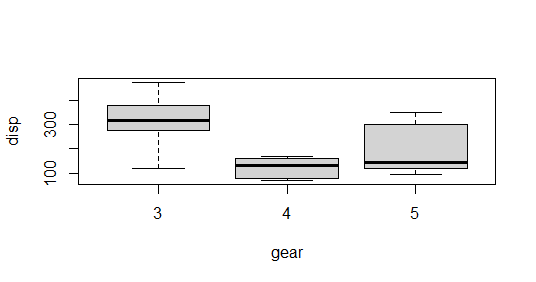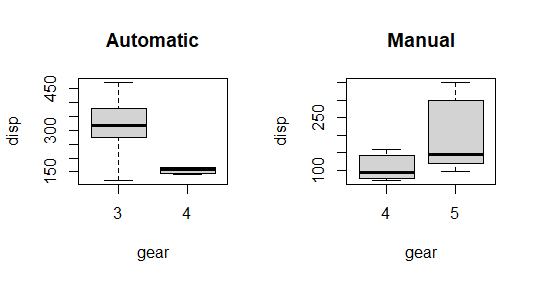# ANOVA Test in R Programming

• Last Updated : 24 Jul, 2020

ANOVA also known as Analysis of variance is used to investigate relations between categorical variable and continuous variable in R Programming. It is a type of hypothesis testing for population variance. ANOVA test involves setting up:

• Null Hypothesis: All population mean are equal.
• Alternate Hypothesis: Atleast one population mean is different from other.

ANOVA test are of two types:

• One way ANOVA: It takes one categorical group into consideration.
• Two way ANOVA: It takes two categorical group into consideration.

#### The Dataset

The mtcars(motor trend car road test) dataset is used which consist of 32 car brands and 11 attributes. The dataset comes preinstalled in `dplyr` package in R.
To get started with ANOVA, we need to install and load the `dplyr` package.

Performing One Way ANOVA test

One way ANOVA test is performed using mtcars dataset which comes preinstalled with dplyr package between disp attribute, a continuous attribute and gear attribute, a categorical attribute.

 `# Installing the package``install.packages(dplyr)``  ` `# Loading the package``library(dplyr)``  ` `# Variance in mean within group and between group``boxplot(mtcars\$disp~factor(mtcars\$gear), ``          ``xlab ``=` `"gear"``, ylab ``=` `"disp"``)``  ` `# Step 1: Setup Null Hypothesis and Alternate Hypothesis``# H0 = mu = mu01 = mu02(There is no difference``# between average displacement for different gear)``# H1 = Not all means are equal``  ` `# Step 2: Calculate test statistics using aov function``mtcars_aov <``-` `aov(mtcars\$disp~factor(mtcars\$gear))``summary(mtcars_aov)``  ` `# Step 3: Calculate F-Critical Value``# For 0.05 Significant value, critical value = alpha = 0.05``  ` `# Step 4: Compare test statistics with F-Critical value ``# and conclude test p < alpha, Reject Null Hypothesis`The box plot shows the mean values of gear with respect of displacement. Hear categorical variable is gear on which factor function is used and continuous variable is disp.The summary shows that gear attribute is very significant to displacement(Three stars denoting it). Also, P value less than 0.05, so it proves that gear is significant to displacement i.e related to each other and we reject the Null Hypothesis.

#### Performing Two Way ANOVA test

Two way ANOVA test is performed using mtcars dataset which comes preinstalled with dplyr package between disp attribute, a continuous attribute and gear attribute, a categorical attribute, am attribute, a categorical attribute.

 `# Installing the package``install.packages(dplyr)``  ` `# Loading the package``library(dplyr)``  ` `# Variance in mean within group and between group``boxplot(mtcars\$disp~mtcars\$gear, subset ``=` `(mtcars\$am ``=``=` `0``), ``         ``xlab ``=` `"gear"``, ylab ``=` `"disp"``, main ``=` `"Automatic"``)``boxplot(mtcars\$disp~mtcars\$gear, subset ``=` `(mtcars\$am ``=``=` `1``),``            ``xlab ``=` `"gear"``, ylab ``=` `"disp"``, main ``=` `"Manual"``)``  ` `# Step 1: Setup Null Hypothesis and Alternate Hypothesis``# H0 = mu0 = mu01 = mu02(There is no difference between``# average displacement for different gear)``# H1 = Not all means are equal``  ` `# Step 2: Calculate test statistics using aov function``mtcars_aov2 <``-` `aov(mtcars\$disp~factor(mtcars\$gear) ``*` `                               ``factor(mtcars\$am))``summary(mtcars_aov2)``  ` `# Step 3: Calculate F-Critical Value``# For 0.05 Significant value, critical value = alpha = 0.05``  ` `# Step 4: Compare test statistics with F-Critical value ``# and conclude test p < alpha, Reject Null Hypothesis`The box plot shows the mean values of gear with respect of displacement. Hear categorical variables are gear and am on which factor function is used and continuous variable is disp.The summary shows that gear attribute is very significant to displacement(Three stars denoting it) and am attribute is not much significant to displacement. P-value of gear is less than 0.05, so it proves that gear is significant to displacement i.e related to each other. P-value of am is greater than 0.05, am is not significant to displacement i.e not related to each other.

#### Results

We see significant results from boxplots and summary.

• Displacement is strongly related to Gears in cars i.e displacement is dependent on gears with p < 0.05.
• Displacement is strongly related to Gears but not related to transmission mode in cars with p 0.05 with am.

My Personal Notes arrow_drop_up